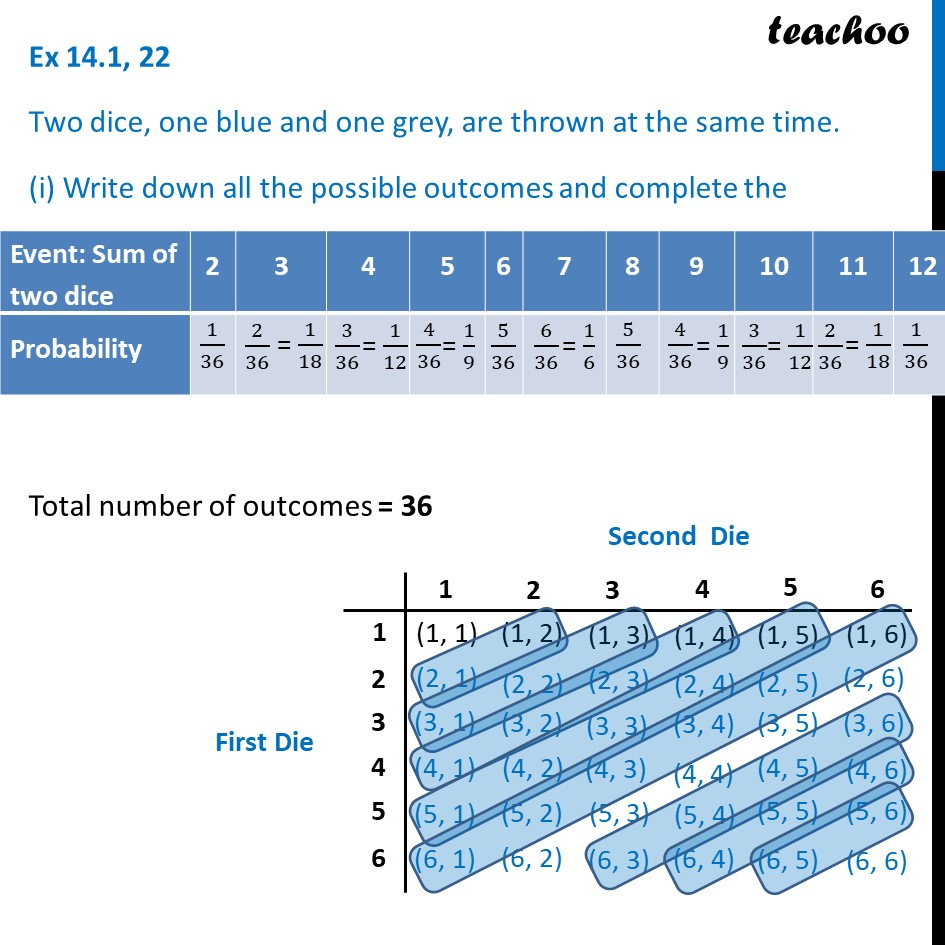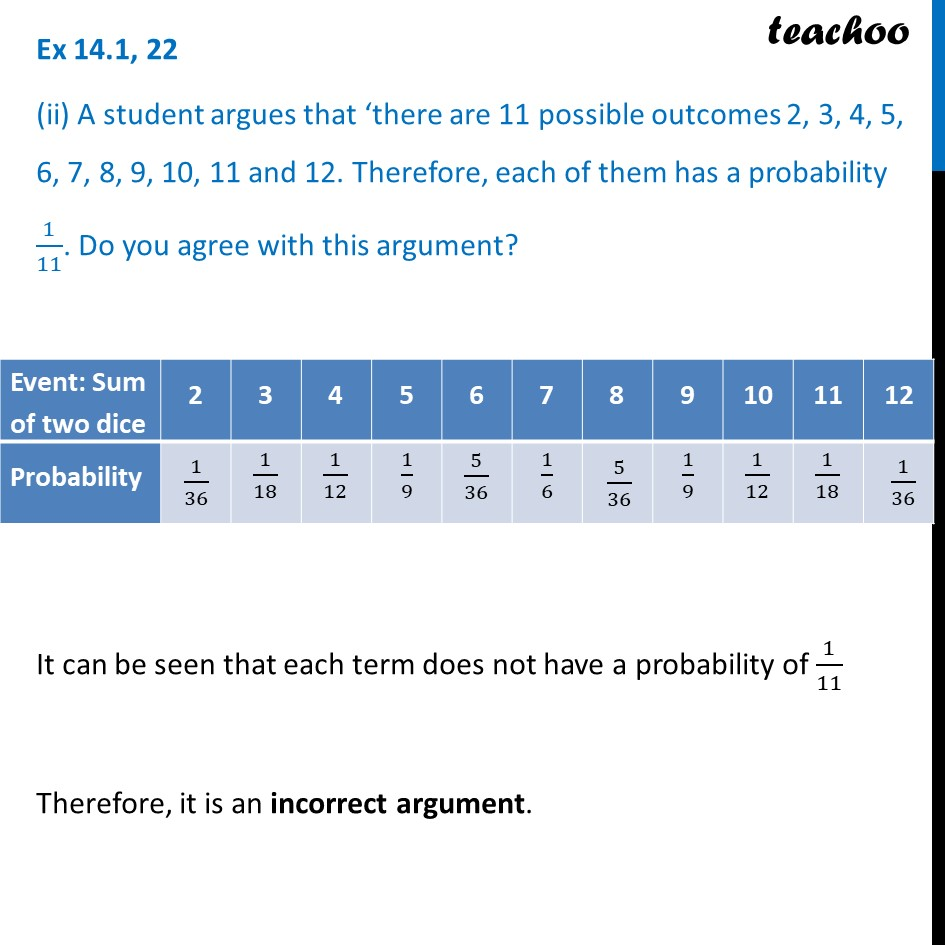Ex 15.1

Chapter 14 Class 10 Probability
Serial order wiseLearn in your speed, with individual attention - Teachoo Maths 1-on-1 Class

### Transcript

Ex 14.1, 22 Two dice, one blue and one grey, are thrown at the same time. (i) Write down all the possible outcomes and complete the following table: Total number of outcomes = 36 Ex 14.1, 22 (ii) A student argues that ‘there are 11 possible outcomes 2, 3, 4, 5, 6, 7, 8, 9, 10, 11 and 12. Therefore, each of them has a probability 1/11. Do you agree with this argument? From the completed table It can be seen that each term does not have a probability of 1/11 Therefore, it is an incorrect argument.